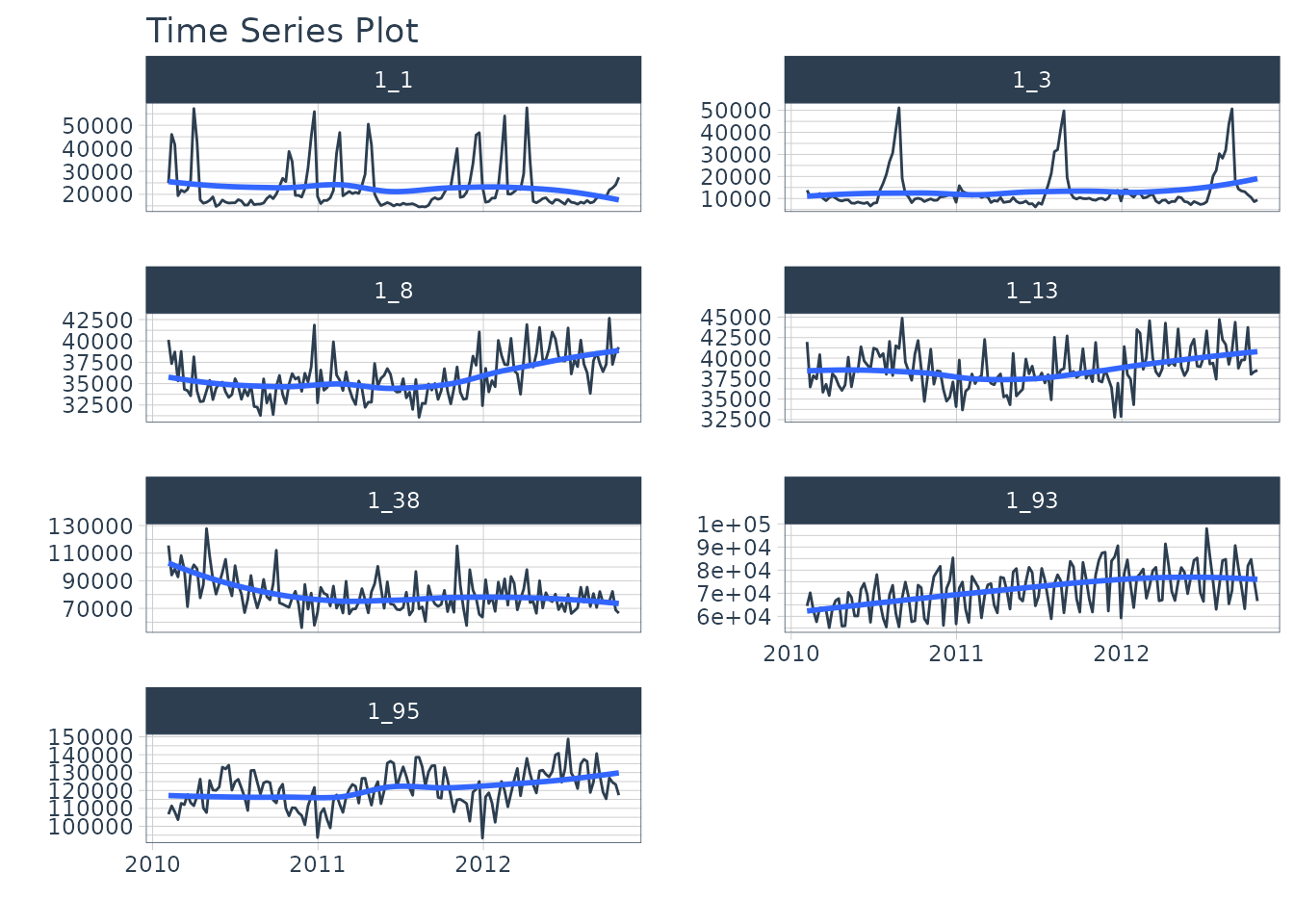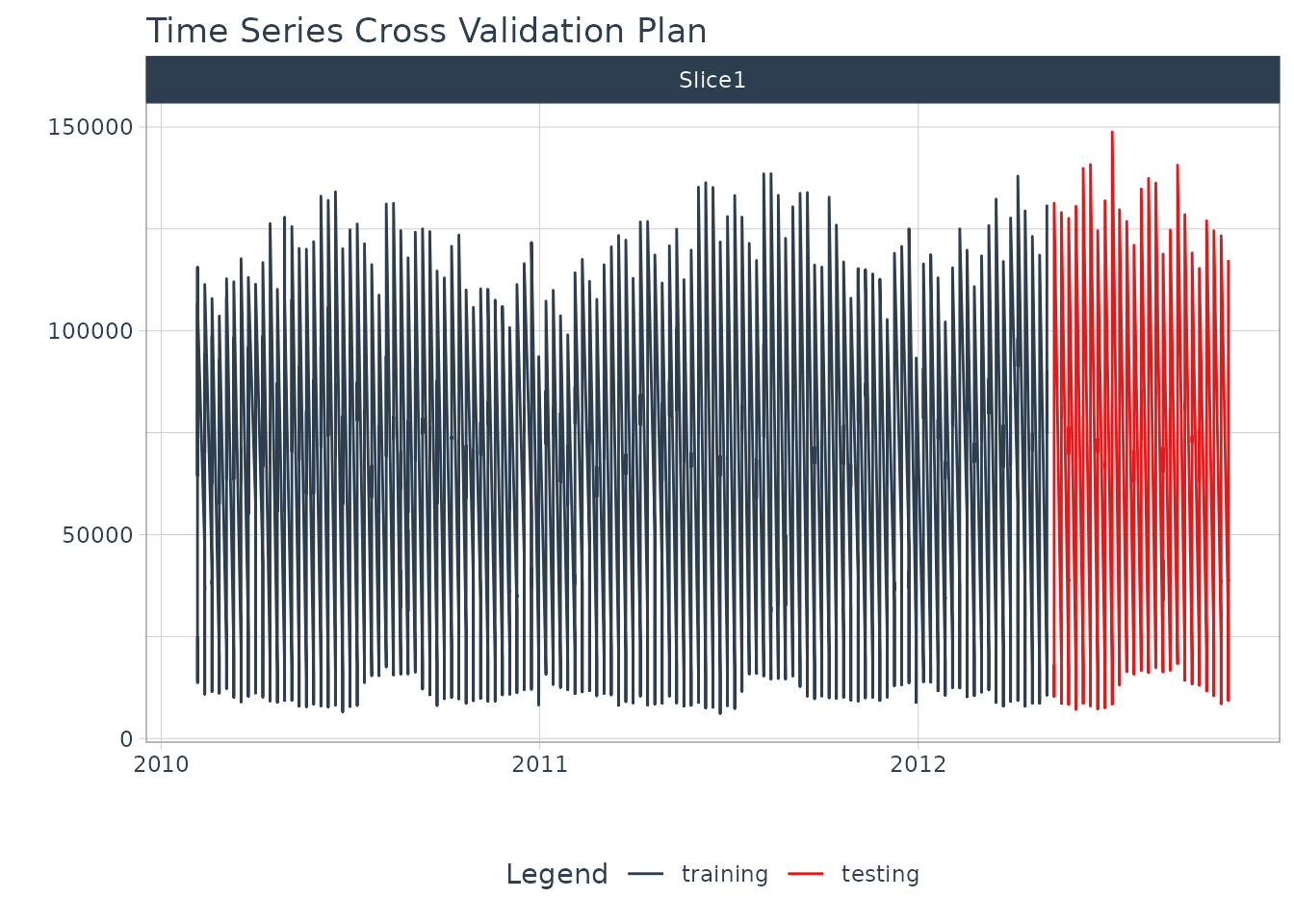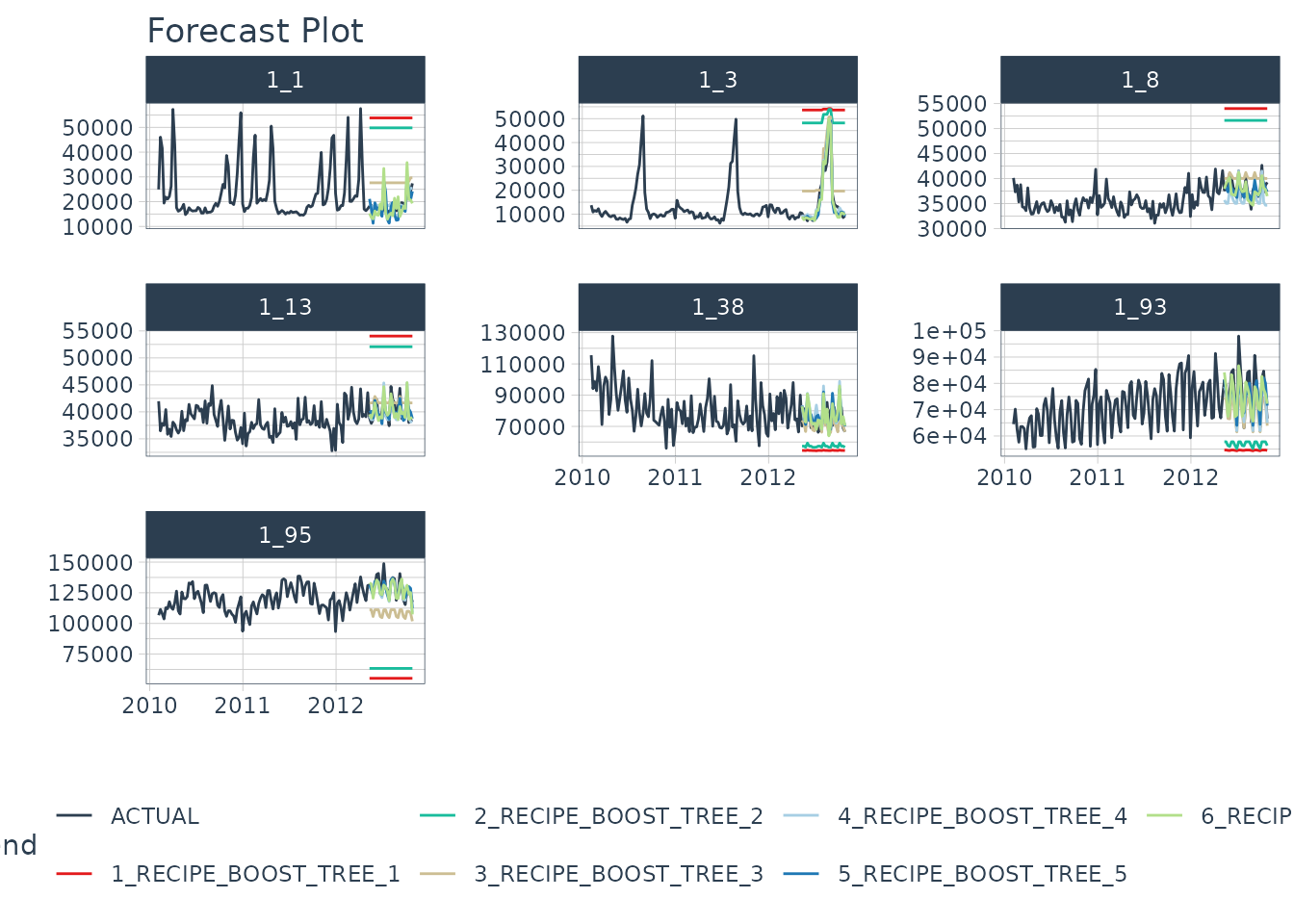Train modeltime models at scale with parallel processing

Fitting many time series models can be an expensive process. To help speed up computation, modeltime now includes parallel processing, which is support for high-performance computing by spreading the model fitting steps across multiple CPUs or clusters.

In this example, we go through a common Hyperparameter Tuning workflow that shows off the modeltime parallel processing integration and support for workflowsets from the tidymodels ecosystem.

## Out-of-the-BoxParallel Processing Functionality Included

The modeltime package (>= 0.6.1) comes with parallel processing functionality.

• Use of parallel_start() and parallel_stop() to simplify the parallel processing setup.

• Use of create_model_grid() to help generating parsnip model specs from dials parameter grids.

• Use of modeltime_fit_workflowset() for initial fitting many models in parallel using workflowsets from the tidymodels ecosystem.

• Use of modeltime_refit() to refit models in parallel.

• Use of control_fit_workflowset() and control_refit() for controlling the fitting and refitting of many models.

## How to Use Parallel Processing

Let’s go through a common Hyperparameter Tuning workflow that shows off the modeltime parallel processing integration and support for workflowsets from the tidymodels ecosystem.

### Libraries

# Machine Learning
library(modeltime)
library(tidymodels)
library(workflowsets)

# Core
library(tidyverse)
library(timetk)

### Setup Parallel Backend

The modeltime package uses parallel_start() to simplify setup, which integrates multiple backend options for parallel processing including:

1. .method = "parallel" (default): Uses the parallel and doParallel packages.

2. .method = "spark": Uses sparklyr. See our tutorial, The Modeltime Spark Backend.

#### Parallel Setup

I’ll set up this tutorial to use two (2) cores using the default parallel package.

• To simplify creating clusters, modeltime includes parallel_start(). We can simply supply the number of cores we’d like to use. To detect how many physical cores you have, you can run parallel::detectCores(logical = FALSE).

• The .method argument specifies that we want the parallel backend.

parallel_start(2, .method = "parallel")

#### Spark Setup

We could optionally run this tutorial with the Spark Backend. For more information, refer to our tutorial The Modeltime Spark Backend.

# OPTIONAL - Run using spark backend
library(sparklyr)

sc <- spark_connect(master = "local")

parallel_start(sc, .method = "spark")

We’ll use the walmart_sales_weeekly dataset from timetk. It has seven (7) time series that represent weekly sales demand by department.

dataset_tbl <- walmart_sales_weekly %>%
select(id, Date, Weekly_Sales)

dataset_tbl %>%
group_by(id) %>%
plot_time_series(
.date_var    = Date,
.value       = Weekly_Sales,
.facet_ncol  = 2,
.interactive = FALSE
)### Train / Test Splits

Use time_series_split() to make a temporal split for all seven time series.

splits <- time_series_split(
dataset_tbl,
assess     = "6 months",
cumulative = TRUE
)

splits %>%
tk_time_series_cv_plan() %>%
plot_time_series_cv_plan(Date, Weekly_Sales, .interactive = F)### Recipe

Make a preprocessing recipe that generates time series features.

recipe_spec_1 <- recipe(Weekly_Sales ~ ., data = training(splits)) %>%
step_timeseries_signature(Date) %>%
step_rm(Date) %>%
step_normalize(Date_index.num) %>%
step_zv(all_predictors()) %>%
step_dummy(all_nominal_predictors(), one_hot = TRUE)

### Model Specifications

We’ll make 6 xgboost model specifications using boost_tree() and the “xgboost” engine. These will be combined with the recipe from the previous step using a workflow_set() in the next section.

#### The general idea

We can vary the learn_rate parameter to see it’s effect on forecast error.

# XGBOOST MODELS
model_spec_xgb_1 <- boost_tree(learn_rate = 0.001) %>%
set_engine("xgboost")

model_spec_xgb_2 <- boost_tree(learn_rate = 0.010) %>%
set_engine("xgboost")

model_spec_xgb_3 <- boost_tree(learn_rate = 0.100) %>%
set_engine("xgboost")

model_spec_xgb_4 <- boost_tree(learn_rate = 0.350) %>%
set_engine("xgboost")

model_spec_xgb_5 <- boost_tree(learn_rate = 0.500) %>%
set_engine("xgboost")

model_spec_xgb_6 <- boost_tree(learn_rate = 0.650) %>%
set_engine("xgboost")

#### A faster way

You may notice that this is a lot of repeated code to adjust the learn_rate. To simplify this process, we can use create_model_grid().

model_tbl <- tibble(
learn_rate = c(0.001, 0.010, 0.100, 0.350, 0.500, 0.650)
) %>%
create_model_grid(
f_model_spec = boost_tree,
engine_name  = "xgboost",
mode         = "regression"
)

model_tbl
#> # A tibble: 6 × 2
#>   learn_rate .models
#>        <dbl> <list>
#> 1      0.001 <spec[+]>
#> 2      0.01  <spec[+]>
#> 3      0.1   <spec[+]>
#> 4      0.35  <spec[+]>
#> 5      0.5   <spec[+]>
#> 6      0.65  <spec[+]>

#### Extracting the model list

We can extract the model list for use with our workflowset next. This is the same result if we would have placed the manually generated 6 model specs into a list().

model_list <- model_tbl\$.models

model_list
#> []
#> Boosted Tree Model Specification (regression)
#>
#> Main Arguments:
#>   learn_rate = 0.001
#>
#> Computational engine: xgboost
#>
#>
#> []
#> Boosted Tree Model Specification (regression)
#>
#> Main Arguments:
#>   learn_rate = 0.01
#>
#> Computational engine: xgboost
#>
#>
#> []
#> Boosted Tree Model Specification (regression)
#>
#> Main Arguments:
#>   learn_rate = 0.1
#>
#> Computational engine: xgboost
#>
#>
#> []
#> Boosted Tree Model Specification (regression)
#>
#> Main Arguments:
#>   learn_rate = 0.35
#>
#> Computational engine: xgboost
#>
#>
#> []
#> Boosted Tree Model Specification (regression)
#>
#> Main Arguments:
#>   learn_rate = 0.5
#>
#> Computational engine: xgboost
#>
#>
#> []
#> Boosted Tree Model Specification (regression)
#>
#> Main Arguments:
#>   learn_rate = 0.65
#>
#> Computational engine: xgboost

### Workflowsets

With the workflow_set() function, we can combine the 6 xgboost models with the 1 recipe to return six (6) combinations of recipe and model specifications. These are currently untrained (unfitted).

model_wfset <- workflow_set(
preproc = list(
recipe_spec_1
),
models = model_list,
cross = TRUE
)

model_wfset
#> # A workflow set/tibble: 6 × 4
#>   wflow_id            info             option    result
#>   <chr>               <list>           <list>    <list>
#> 1 recipe_boost_tree_1 <tibble [1 × 4]> <opts> <list >
#> 2 recipe_boost_tree_2 <tibble [1 × 4]> <opts> <list >
#> 3 recipe_boost_tree_3 <tibble [1 × 4]> <opts> <list >
#> 4 recipe_boost_tree_4 <tibble [1 × 4]> <opts> <list >
#> 5 recipe_boost_tree_5 <tibble [1 × 4]> <opts> <list >
#> 6 recipe_boost_tree_6 <tibble [1 × 4]> <opts> <list >

### Parallel Training (Fitting)

We can train each of the combinations in parallel.

#### Controlling the Fitting Proces

Each fitting function in modeltime has a “control” function:

• control_fit_workflowset() for modeltime_fit_workflowset()
• control_refit() for modeltime_refit()

The control functions help the user control the verbosity (adding remarks while training) and set up parallel processing. We can see the output when verbose = TRUE and allow_par = TRUE.

• allow_par: Whether or not the user has indicated that parallel processing should be used.

• If the user has set up parallel processing externally, the clusters will be reused.

• If the user has not set up parallel processing, the fitting (training) process will set up parallel processing internally and shutdown. Note that this is more expensive, and usually costs around 10-15 seconds to set up.

• verbose: Will return important messages showing the progress of the fitting operation.

• cores: The cores that the user has set up. Since we’ve already set up doParallel to use 2 cores, the control recognizes this.

• packages: The packages are packages that will be sent to each of the workers.

control_fit_workflowset(
verbose   = TRUE,
allow_par = TRUE
)
#> workflowset control object
#> --------------------------
#> allow_par : TRUE
#> cores     : 2
#> verbose   : TRUE
#> packages  : modeltime parsnip workflows dplyr stats lubridate tidymodels timetk rsample recipes yardstick dials tune forcats stringr readr tidyverse workflowsets tidyr tibble purrr modeldata infer ggplot2 scales broom graphics grDevices utils datasets methods base

#### Fitting Using Parallel Backend

We use the modeltime_fit_workflowset() and control_fit_workflowset() together to train the unfitted workflowset in parallel.

model_parallel_tbl <- model_wfset %>%
modeltime_fit_workflowset(
data    = training(splits),
control = control_fit_workflowset(
verbose   = TRUE,
allow_par = TRUE
)
)
#> Using existing parallel backend with 2 clusters (cores)...
#>  Beginning Parallel Loop | 0.013 seconds
#>  Finishing parallel backend. Clusters are remaining open. | 17.534 seconds
#>  Close clusters by running: parallel_stop().
#>  Total time | 17.535 seconds

This returns a modeltime table.

model_parallel_tbl
#> # Modeltime Table
#> # A tibble: 6 × 3
#>   .model_id .model     .model_desc
#>       <int> <list>     <chr>
#> 1         1 <workflow> RECIPE_BOOST_TREE_1
#> 2         2 <workflow> RECIPE_BOOST_TREE_2
#> 3         3 <workflow> RECIPE_BOOST_TREE_3
#> 4         4 <workflow> RECIPE_BOOST_TREE_4
#> 5         5 <workflow> RECIPE_BOOST_TREE_5
#> 6         6 <workflow> RECIPE_BOOST_TREE_6

#### Comparison to Sequential Backend

We can compare to a sequential backend. We have a slight perfomance boost. Note that this performance benefit increases with the size of the training task.

model_sequential_tbl <- model_wfset %>%
modeltime_fit_workflowset(
data    = training(splits),
control = control_fit_workflowset(
verbose   = TRUE,
allow_par = FALSE
)
)
#> ℹ Fitting Model: 1
#> ✔ Model Successfully Fitted: 1
#> ℹ Fitting Model: 2
#> ✔ Model Successfully Fitted: 2
#> ℹ Fitting Model: 3
#> ✔ Model Successfully Fitted: 3
#> ℹ Fitting Model: 4
#> ✔ Model Successfully Fitted: 4
#> ℹ Fitting Model: 5
#> ✔ Model Successfully Fitted: 5
#> ℹ Fitting Model: 6
#> ✔ Model Successfully Fitted: 6
#> Total time | 24.663 seconds

### Accuracy Assessment

We can review the forecast accuracy. We can see that Model 5 has the lowest MAE.

model_parallel_tbl %>%
modeltime_calibrate(testing(splits)) %>%
modeltime_accuracy() %>%
table_modeltime_accuracy(.interactive = FALSE)
.model_id .model_desc .type mae mape mase smape rmse rsq Accuracy Table 1 RECIPE_BOOST_TREE_1 Test 55572.50 98.52 1.63 194.17 66953.92 0.96 2 RECIPE_BOOST_TREE_2 Test 48819.23 86.15 1.43 151.49 58992.30 0.96 3 RECIPE_BOOST_TREE_3 Test 13286.28 21.41 0.39 24.70 17271.50 0.98 4 RECIPE_BOOST_TREE_4 Test 3802.13 8.11 0.11 8.05 5558.56 0.98 5 RECIPE_BOOST_TREE_5 Test 3361.45 7.01 0.10 7.08 5233.28 0.98 6 RECIPE_BOOST_TREE_6 Test 3645.01 8.76 0.11 9.20 5420.95 0.98

### Forecast Assessment

We can visualize the forecast.

model_parallel_tbl %>%
modeltime_forecast(
new_data    = testing(splits),
actual_data = dataset_tbl,
keep_data   = TRUE
) %>%
group_by(id) %>%
plot_modeltime_forecast(
.facet_ncol  = 3,
.interactive = FALSE
)### Closing Clusters

We can close the parallel clusters using parallel_stop().

parallel_stop()

## Summary

We just showcased a simple Hyperparameter Tuning example using Parallel Processing. But this is a simple problem. And, there’s a lot more to learning time series.

• Many more algorithms
• Ensembling
• Machine Learning
• Deep Learning
• Scalable Modeling: 10,000+ time series

Your probably thinking how am I ever going to learn time series forecasting. Here’s the solution that will save you years of struggling.

### Take the High-Performance Forecasting Course

Become the forecasting expert for your organizationHigh-Performance Time Series Course

#### Time Series is Changing

Time series is changing. Businesses now need 10,000+ time series forecasts every day. This is what I call a High-Performance Time Series Forecasting System (HPTSF) - Accurate, Robust, and Scalable Forecasting.

High-Performance Forecasting Systems will save companies by improving accuracy and scalability. Imagine what will happen to your career if you can provide your organization a “High-Performance Time Series Forecasting System” (HPTSF System).

#### How to Learn High-Performance Time Series Forecasting

I teach how to build a HPTFS System in my High-Performance Time Series Forecasting Course. You will learn:

• Time Series Machine Learning (cutting-edge) with Modeltime - 30+ Models (Prophet, ARIMA, XGBoost, Random Forest, & many more)
• Deep Learning with GluonTS (Competition Winners)
• Time Series Preprocessing, Noise Reduction, & Anomaly Detection
• Feature engineering using lagged variables & external regressors
• Hyperparameter Tuning
• Time series cross-validation
• Ensembling Multiple Machine Learning & Univariate Modeling Techniques (Competition Winner)
• Scalable Forecasting - Forecast 1000+ time series in parallel
• and more.

Become the Time Series Expert for your organization.